Concept of Blurring

A brief introduction of blurring has been discussed in our previous tutorial of concept of masks, but we are formally going to discuss it here.

Blurring

In blurring, we simple blur an image. An image looks more sharp or more detailed if we are able to perceive all the objects and their shapes correctly in it. For example. An image with a face, looks clear when we are able to identify eyes, ears, nose, lips, forehead e.t.c very clear. This shape of an object is due to its edges. So in blurring, we simple reduce the edge content and makes the transition form one color to the other very smooth.

Blurring vs zooming

You might have seen a blurred image when you zoom an image. When you zoom an image using pixel replication, and zooming factor is increased, you saw a blurred image. This image also has less details, but it is not true blurring.

Because in zooming, you add new pixels to an image, that increase the overall number of pixels in an image, whereas in blurring, the number of pixels of a normal image and a blurred image remains the same.

Common example of a blurred image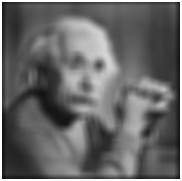Types of filters

Blurring can be achieved by many ways. The common type of filters that are used to perform blurring are.

• Mean filter
• Weighted average filter
• Gaussian filter

Out of these three, we are going to discuss the first two here and Gaussian will be discussed later on in the upcoming tutorials.

Mean filter

Mean filter is also known as Box filter and average filter. A mean filter has the following properties.

• It must be odd ordered
• The sum of all the elements should be 1
• All the elements should be same

If we follow this rule, then for a mask of 3x3. We get the following result.

 1/9 1/9 1/9 1/9 1/9 1/9 1/9 1/9 1/9

Since it is a 3x3 mask, that means it has 9 cells. The condition that all the element sum should be equal to 1 can be achieved by dividing each value by 9. As

1/9 + 1/9 + 1/9 + 1/9 + 1/9 + 1/9 + 1/9 + 1/9 + 1/9 = 9/9 = 1

Original Image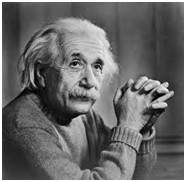Blurred Image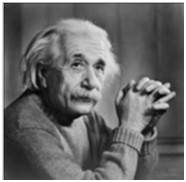May be the results are not much clear. Let’s increase the blurring. The blurring can be increased by increasing the size of the mask. The more is the size of the mask, the more is the blurring. Because with greater mask, greater number of pixels are catered and one smooth transition is defined.

Original ImageBlurred Image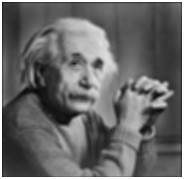Same way if we increase the mask, the blurring would be more and the results are shown below.

Original ImageBlurred Image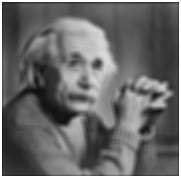Original ImageBlurred Image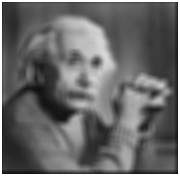Original ImageBlurred Image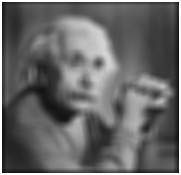Weighted average filter

In weighted average filter, we gave more weight to the center value. Due to which the contribution of center becomes more then the rest of the values. Due to weighted average filtering, we can actually control the blurring.

Properties of the weighted average filter are.

• It must be odd ordered
• The sum of all the elements should be 1
• The weight of center element should be more then all of the other elements

Filter 1

 1 1 1 1 2 1 1 1 1

The two properties are satisfied which are (1 and 3). But the property 2 is not satisfied. So in order to satisfy that we will simple divide the whole filter by 10, or multiply it with 1/10.

Filter 2

 1 1 1 1 10 1 1 1 1

Dividing factor = 18.